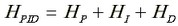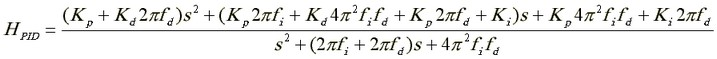﻿ 20-sim webhelp > Toolboxes > Control Toolbox > Filter Editor > Controllers > PID-Controller

# PID-Controller

The PID Controller consists of an integrator and differentiator with proportional gain.

 • Gain Kp: The controller gain Kp determines the frequency gain of the controller in the pass band (where integration and differentiation are of no interest).
 • Gain Ki: The controller gain Ki determines the shape of the frequency response at low frequencies.
 • Gain Kd: The controller gain Kp determines the shape of the frequency response at high frequencies.
 • Frequency fi: The integration behavior of the controller starts at frequency fi in Hz. A decrease of this frequency corresponds to a more pure integration behavior.
 • Frequency fd: The differentiation behavior of the controller stops at frequency fd in Hz. An increase of this frequency corresponds to a more pure differentiation behavior, reducing the tameness of the controller.

Transfer function of the controller:that equals